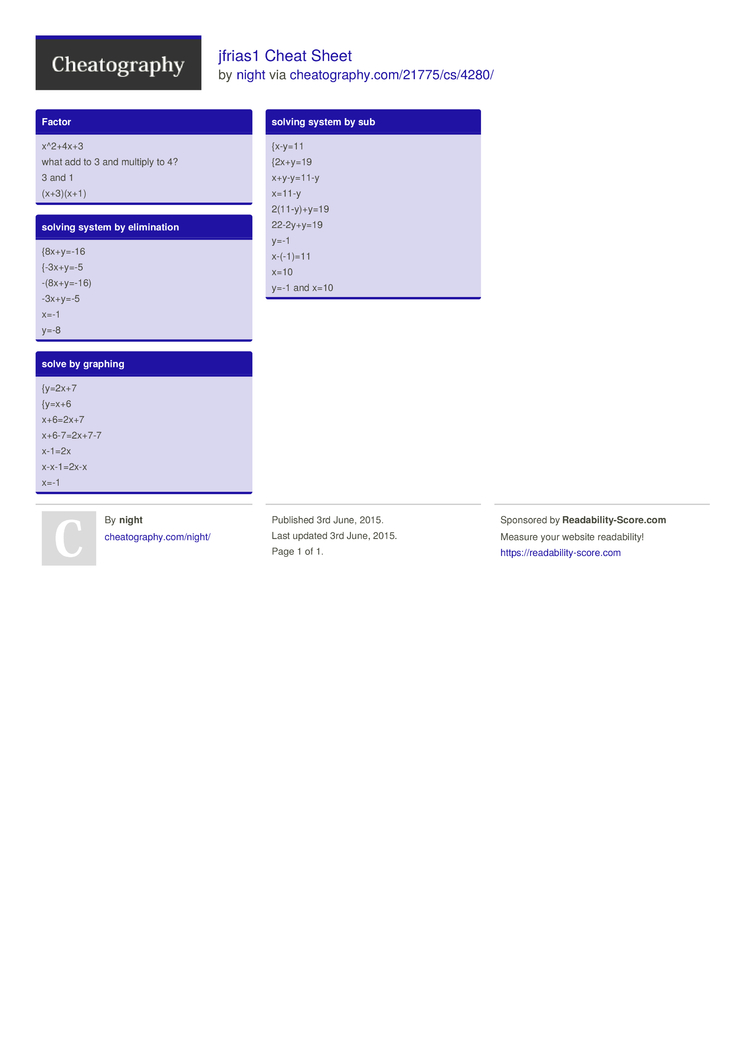# jfrias1 Cheat Sheet by night

### Factor

 x^2+4x+3 what add to 3 and multiply to 4? 3 and 1 (x+3)(x+1)

### solving system by elimin­ation

 {8x+y=-16 {-3x+y=-5 -(8x+y­=-16) -3x+y=-5 x=-1 y=-8

### solve by graphing

 {y=2x+7 {y=x+6 x+6=2x+7 x+6-7=­2x+7-7 x-1=2x x-x-1=2x-x x=-1

### solving system by sub

 {x-y=11 {2x+y=19 x+y-y=11-y x=11-y 2(11-y­)+y=19 22-2y+y=19 y=-1 x-(-1)=11 x=10 y=-1 and x=101 Page
//media.cheatography.com/storage/thumb/night_jfrias1.750.jpg

PDF (recommended)